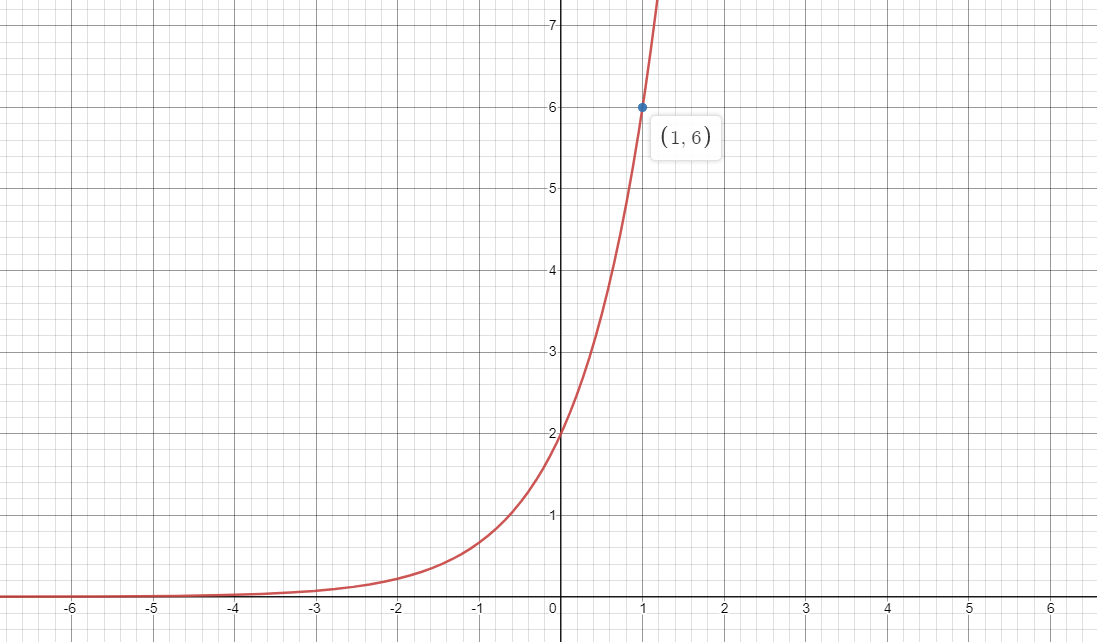# Help Zone

### Student Question

Hello,

I am having difficulty finding the equation of an exponential function. Could you help me please?

(Maths CST4)

Thank you very much!

Mathematics{t c="richEditor.description.title"} {t c="richEditor.description.paragraphMenu"} {t c="richEditor.description.inlineMenu"} {t c="richEditor.description.embed"}

## Explanations (1)

•Explanation from AlloprofExplanation from Alloprof

This Explanation was submitted by a member of the Alloprof team.

Hi TomateSupra7536,

Thanks for your question! 😉

Before starting, it is important to remember the equation of the exponential function and the role of each factor. We usually find the exponential function in this form:

y = a (c) x

The parameter a corresponds to the y-intercept and its sign defines whether the curve is open upwards or downwards. The parameter c is the growth factor, if it is greater than 1, the function is increasing and if it is between 0 and 1, the function is decreasing.

When it comes to finding the equation of an exponential function, there are two techniques.

• If the y-intercept is given

Let us take this graph as an example:If the y-intercept is given, here (0,2), it corresponds to the value of the parameter a. So in this equation, the parameter a is 2. Then, we have to use the second point to find the parameter c. We then obtain this:

6 = 2 (c) 1

If we isolate the variable c, we obtain c = 3. The equation of this graph is therefore:

y = 2 (3) x

• If the y-intercept is not given

If we take the previous graph and we only have the coordinates (1,6) and (2,18), what can we do if we don't have the parameter a? It is necessary to make a system of equations with the coordinates which are given. We thus obtain this:

6= a (c) 1

18 = a (c) 2

If we manipulate the equations, it is possible to use the comparative method to find the parameter c. If we isolate a in the two equations, we get this:

6c = a

18c2 = a

After some calculations, we get c = 2. It only remains to find the parameter a, as with the first technique, using one of the equations and one of the coordinates to obtain the equation of the function.